# CBSEtips.in

## Thursday, 28 January 2021

### CBSE Class 10 Maths - MCQ Questions and Online Tests - Unit 6 - Triangles

#### CBSE Class 10 Maths – MCQ and Online Tests – Unit 6 – Triangles

Every year CBSE conducts board exams for 10th standard. These exams are very competitive to all the students. So our website provides online tests for all the 10th subjects. These tests are also very effective and useful for those who preparing for competitive exams like NEET, JEE, CA etc. It can boost their preparation level and confidence level by attempting these chapter wise online tests.

These online tests are based on latest CBSE Class 10 syllabus. While attempting these our students can identify the weak lessons and continuously practice those lessons for attaining high marks. It also helps to revise the NCERT textbooks thoroughly.

#### CBSE Class 10 Maths – MCQ and Online Tests – Unit 6 – Triangles

Question 1.
In the given figure, ∠T and ∠B are right angles. If the length of AT, BC and AS (in centimeters) are 15, 16, and 17 respectively, then the length of TC (in centimeters) is: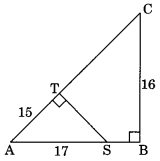(a) 18
(b) 16
(c) 19
(d) 12

Question 2.
In the given figure, value of x(in cm) is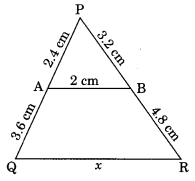(a) 4
(b) 5
(c) 6
(d) 8

Question 3.
In the given figure ΔABC ~ ΔPQR. The value of x is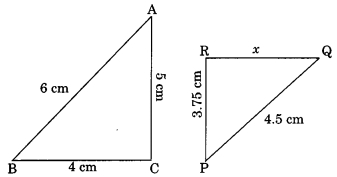(a) 2.5 cm
(b) 3.5 cm
(c) 2.75 cm
(d) 3 cm

Question 4.
In ΔABC, if DE || BC, AD = x, DB = x – 2, AE = x + 2 and EC = x – 1, then value of x is
(a) 3
(b) 4
(c) 5
(d) 3.5

Question 5.
The perimeters of two similar triangles ABC and PQR are 60 cm and 36 cm respectively. If PQ = 9 cm, then AB equals
(a) 6 cm
(b) 10 cm
(c) 15 cm
(d) 24 cm

Question 6.
If ΔABC is similar to ΔDEF such that 2 AB = DE and BC = 8 cm then EF is equal to.
(a) 12 cm
(b) 4 cm
(c) 16 cm
(d) 8 c

Question 7.
In ΔABC, AB = 6 cm and DE || BC such that AE = $$\frac{1}{4}$$ AC then the length of AD is
(a) 2 cm
(b) 1.2 cm
(c) 1.5 cm
(d) 4 cm

Question 8.
In the given figure DE || AC which of the following is true?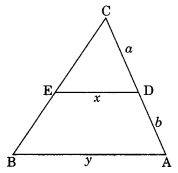(a) x = $$\frac{a+b}{ay}$$
(b) y = $$\frac{ax}{a+b}$$
(c) x = $$\frac{ay}{a+b}$$
(d) $$\frac{x}{y}$$ = $$\frac{a}{b}$$

Answer: (c) x = $$\frac{ay}{a+b}$$

Question 9.
ΔABC ~ ΔDEF. If AB = 4 cm, BC = 3.5 cm, CA = 2.5 cm and DF = 7.5 cm, then the perimeter of ΔDEF is
(a) 10 cm
(b) 14 cm
(c) 30 cm
(d) 25 cm

Question 10.
In the figure PQ || BC. If $$\frac{PQ}{BC}$$ = $$\frac{2}{5}$$ then $$\frac{AP}{PB}$$ is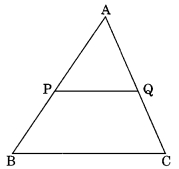(a) $$\frac{2}{5}$$
(b) $$\frac{2}{3}$$
(c) $$\frac{3}{2}$$
(c) $$\frac{3}{5}$$

Answer: (b) $$\frac{2}{3}$$

Question 11.
In the given figure, ΔACB ~ ΔAPQ. If AB = 6 cm, BC = 8 cm, and PQ = 4 cm then AQ is equal to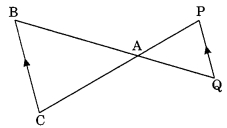(a) 2 cm
(b) 2.5 cm
(c) 3 cm
(d) 3.5 cm

Question 12.
ΔDEF ~ ΔABC. If DE : AB = 2 : 3 and ar ΔDEF is equal to 44 square units then ar (ΔABC) (square unit) is
(a) 99
(b) 120
(c) $$\frac{176}{9}$$
(d) 66

Question 13
ΔABC and ΔBDE are two equilateral triangles such that D is the mid point of BC. Ratio of the areas of triangle ΔABC and ΔBDE is.
(a) 2 : 1
(b) 1 : 2
(c) 4 : 1
(d) 1 : 4

Question 14.
If ΔABC ~ ΔPQR, $$\frac{ar ΔABC}{ar ΔPQR}$$ = $$\frac{9}{4}$$ and AB = 18 cm, then the length of PQ is
(a) 14 cm
(b) 8 cm
(c) 10 cm
(d) 12 cm

Question 15.
In the given figure ΔABC ~ ΔPQR, PM is median of ΔPQR. If ar ΔABC = 289 cm², BC = 17 cm, MR = 6.5 cm then the area of ΔPQM is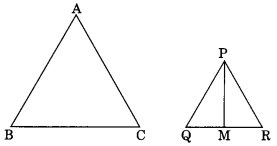(a) 169 cm²
(b) 13 cm²
(c) 84.5 cm²
(d) 144.5 cm²

Question 16.
If the ratio of the perimeters of two similar triangles is 4 : 25, then the ratio of the areas of the similar triangles is
(a) 16 : 625
(b) 2 : 5
(c) 5 : 2
(d) 625 : 16

Question 17.
In the given figure, PQ = 24 cm, QR = 26 cm ∠PAR = 90°, PA = 6 cm, and AR = 8 cm, the degree measure of ∠QPR is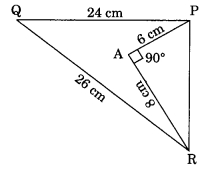(a) 90°
(b) 100°
(c) 50°
(d) 45°

Question 18.
In the given figure the value of x is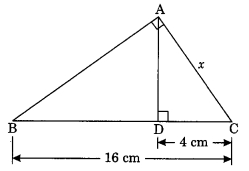(a) 4 cm
(b) 5 cm
(c) 8 cm
(d) 3 cm

Question 19.
ΔPQR is an equilateral triangle with each side of length 2p. If PS ⊥ QR, then PS is equal to
(a) $$\frac{√3}{2}$$p
(b) 2p
(c) √3p
(d) p

Question 20.
In ΔLMN, ∠L = 50° and ∠N = 60°, If ΔLMN ~ ΔPQR, then find ∠Q
(a) 50°
(b) 70°
(c) 60°
(d) 40°

Share: